## Example Questions

### Example Question #1 : Permutation / Combination

A chamber of commerce board has seven total members, drawn from a pool of twenty candidates.   There are two stages in the board's election process.  First, a president, secretary, and treasurer are chosen.  After that, four members are chosen to be “at large” without any specific title or district.  How many possible boards could be chosen?

16,279,200

390,700,800

2,713,200

10,465,200

5,426,400

16,279,200

Explanation:

We have to consider two cases.  First, the group containing the president, secretary, and treasurer represents a case of permutation.  Since the order matters in such a group, we can select from our initial 20 candidates 20 * 19 * 18, or 6840 possible groupings.

Following that, the at large group constitutes a case of combinations in which the order does not matter.  Since we have chosen 3 already for the first three slots, there will be 17 remaining people.  The formula for choosing 4-person sets out of 17 candidates is represented by the combination formula of this form:

17! / ((17-4)! * 4!) = 17! / (13! * 4!) = (17 * 16 * 15 * 14) / (4 * 3 * 2) = 17 * 4 * 5 * 7 = 2380

Thus, we have 6840 and 2380 possible groupings.  These can each be combined with each other, meaning that we have 6840  * 2380, or 16,279,200 potential boards.

### Example Question #1 : Permutation / Combination

How many ordered samples of 5 cards can be drawn from a deck of 52 cards without replacement?

11,452,000

42,365,000

65,690,040

311,875,200

500,320,000

311,875,200

Explanation:

The key points we need to remember are that order matters and that we are sampling without replacement.  This then becomes a simple permutation problem.  We have 52 cards to be chosen 5 at a time, so the answer is 52 * 51 * 50 * 49 * 48.

### Example Question #1 : Permutation / Combination

Quantitative Comparison

3 cards are chosen from a standard 52-card deck.

Quantity A: The number of ways to choose 3 cards with replacement

Quantity B: The number of ways to choose 3 cards without replacement

The two quantities are equal.

Quantity B is greater.

The relationship cannot be determined from the information given.

Quantity A is greater.

Quantity A is greater.

Explanation:

Quantity A says with replacement, so we have 52 ways to choose the first card, then we replace it so we again have 52 ways to choose the 2nd card, and similarly we have 52 ways to choose the 3rd card.  Therefore we have 52 * 52 * 52 ways of choosing 3 cards with replacement.

Quantity B says without replacement, so we have 52 ways to choose the first card, but then we don't put that card back in the deck so we have 51 ways to choose the second card. Again we don't put that card back, leaving 50 ways to choose the third card. Thus there are 52 * 51 * 50 ways of choosing 3 cards without replacement.

Clearly Quantity A is greater. In general, there should always be more ways to choose something with replacement than there are without replacement just as we showed above. If you already knew this, you could have picked Quantity A without the math. Notice that either way you come to the answer here, there is NO reason to finish the computations all the way through. This will save you time on many quantitative comparison problems. For example, we know that 52 * 52 * 52 is larger than 52 * 51 * 50 without actually figuring out what the two expressions equal.

### Example Question #1 : Permutation / Combination

In how many ways can 6 differently colored rose bushes be planted in a row that only has room for 4 bushes?

480

240

120

220

360

360

Explanation:

There are 6 ways to choose the first rose bush, 5 ways to choose the second, 4 ways to choose the third, and 3 ways to choose the fourth. In total there are 6 * 5 * 4 * 3 = 360 ways to arrange the rose bushes.

### Example Question #1 : How To Find Permutation Notation

In how many ways can five different colored balls be arranged in a row?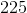Explanation:

We need to arrange the 5 balls in 5 positions: _ _ _ _ _.  The first position can be taken by any of the 5 balls. Then there are 4 balls left to fill the second position, and so on.  Therefore the number of arrangements is 5! = 5 * 4 * 3 * 2 * 1 = 120.

### Example Question #2 : Permutation / Combination

Daisy wants to arrange four vases in a row outside of her garden. She has eight vases to choose from. How many vase arrangements can she make?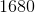Explanation:

For this problem, since the order of the vases matters (red blue yellow is different than blue red yellow), we're dealing with permutations.

Withselections made from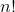potential options, the total number of possible permutations(order matters) is: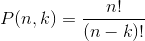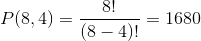Tired of practice problems?

Try live online GRE Math prep today.